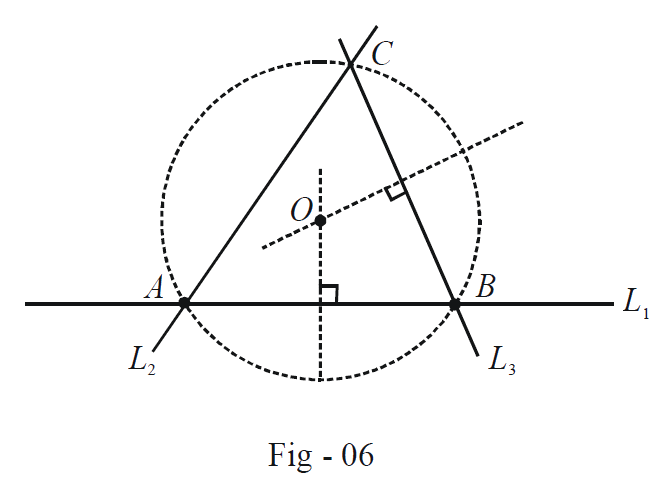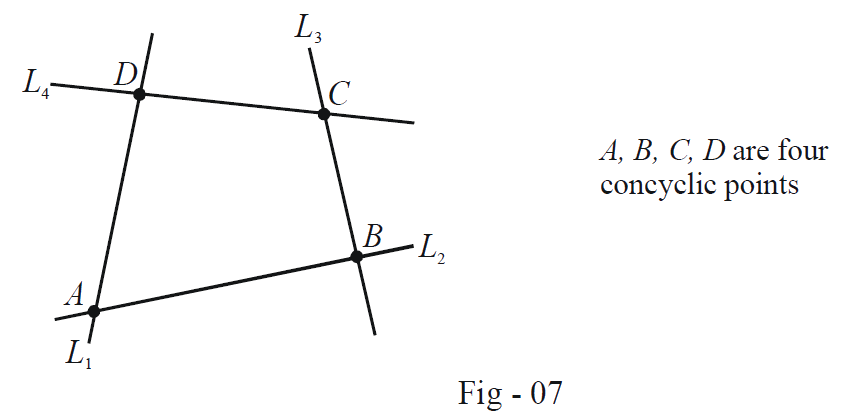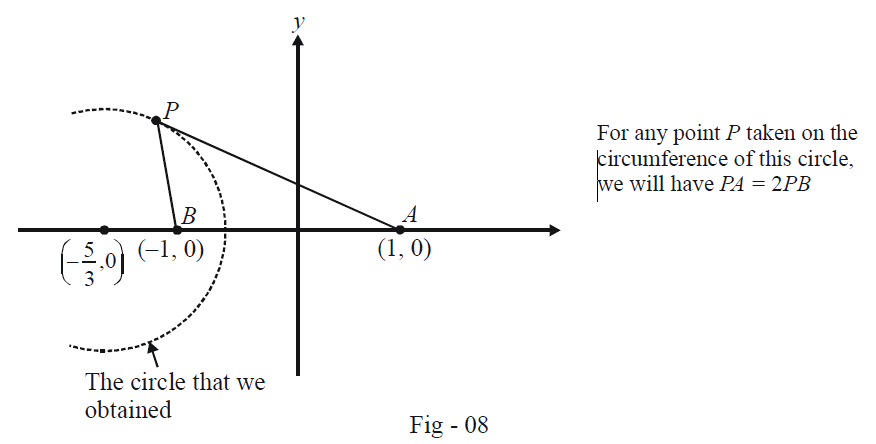# Jee Examples On Equations Of Circles Set-1

Go back to  'Circles'

Example -7

Find the equation of the circle circumscribing the triangle formed by the lines \begin{align}x + y = 6,\,\,2x + y = 4\,\,\,{\text{and}}\,\,x + 2y = 5\end{align}.

Solution: Let us first consider the general case wherein we’ve been given three lines $$L_1, L_2$$ and $$L_3$$ and we need to find the circle circumscribing the triangle that these three lines formOne way to do it would be as follows:

• Find the intersection points $$A, B$$ and $$C$$ of the three lines
• Use these intersection points to write any two perpendicular bisectors
• Find the intersection of these two perpendicular bisectors which gives us the center $$O$$ .
• Finally, find the radius (which will equal $$OA, OB$$ and $$OC$$)

This procedure will definitely become quite lengthy. We look instead for a a more elegant method.

We first try to write the equation of an arbitrary second-degree curve S passing through the intersection points of $$L_1, L_2$$ and $$L_3$$. Think carefully and you’ll realize that such a curve can be written in terms of two arbitrary constants and as follows:

\begin{align}S \equiv {L_1}{L_2} + \lambda \,{L_2}{L_3} + \mu \,{L_3}{L_1} = 0\end{align}

That such a curve $$S$$ will pass through all the three intersection points can be verified by observing that the substitution of the co-ordinates of any of the three points in the equation above will make both sides identically $$0$$.

One we have such a curve, we can impose the necessary constraints to make it a circle.

Coming back to the current example, the equation of an arbitrary curve passing through the intersection points of the three lines can be written as:

\begin{align}S \equiv \left( {x + y - 6} \right)\left( {2x + y - 4} \right) + \lambda \left( {2x + y - 4} \right)\left( {x + 2y - 5} \right) + \mu \left( {x + 2y - 5} \right)\left( {x + y - 6} \right) = 0\end{align}

To make $$S$$ the equation of a circle, we simple impose the following constraints:

\begin{align} {\bf{Coeff}}{\bf{.}}\,\,{\bf{of}}\,{x^2} = {\bf{Coeff}}{\bf{.}}\,\,{\bf{of}}\,{y^{\bf{2}}} \quad & \Rightarrow \qquad 2 + 2\lambda + \mu = 1 + 2\lambda + 2\mu \\ & \Rightarrow \qquad \mu = 1 \qquad \qquad \qquad \qquad \qquad ...(1)\\ {\bf{Coeff}}{\bf{.}}\,\,{\bf{of}}\,xy{\bf{ = 0}} \qquad \qquad \qquad \, & \Rightarrow \qquad 3 + 5\lambda + 3\mu = 0\\ & \Rightarrow \qquad \lambda = - \frac{6}{5} \qquad \qquad \qquad \qquad \quad ...(2) \end{align}

We substitute \begin{align}\lambda \;{\rm{and}}\;\mu \end{align} back into $$S$$ to obtain the required equation as:

\begin{align}S \equiv {x^2} + {y^2} - 17x - 19y + 50 = 0\end{align}

As another example of following such an approach, suppose that we are given four straight lines and are told that they intersect at four concyclic points, as shown below:What approach will you follow if you’re told to find the equation of the circle circumscribing this quadrilateral? Obviously, one can always proceed by explicitly determining the centre and the radius of the said circle, but as in the previous question, a much more elegant method exists.

Convince yourself that any second degree curve S passing through $$A, B, C, D$$ can we written as

$S \equiv {L_1}{L_3} + \lambda \,{L_2}{L_4} = 0$

Observe carefully that the substitution of the co-ordinates of any of the four points $$A, B, C, D$$ will make both sides identically $$0$$, implying that these four points lie on $$S$$. We now simply impose the necessary constraint \begin{align}\left( {{\rm{on}}\,\lambda } \right)\end{align} to make S represent a circle, thus obtaining $$S!$$

Example -8

Consider a circle of radius $$r$$ centred at the origin:

${x^2} + {y^2} = {r^2}$

A line $$y = mx + c$$ either just touches this circle or intersects it in two distinct points. What condition must $$m$$ and $$c$$ satisfy?

Solution:    What we need to do algebraically is solve the simultaneous system of equations

\begin{align} {x^2} + {y^2} = {r^2} & & & ...(1)\\ y = mx + c & & & ...(2) \end{align}

and find the condition on $$m$$ and $$c$$ for this system to have two distinct roots.

We substitute the value of $$y$$ from (2) in (1):

\begin{align} & {x^2} + {\left( {mx + c} \right)^2} = {r^2}\\ \Rightarrow \qquad & \left( {1 + {m^2}} \right){x^2} + 2mc\,x + {c^2} - {r^2} = 0 & & ...(3) \end{align}

Since the line intersects the circle (or touches it) the discriminant of (3) cannot be non-negative since at least one real value of $$x$$ must exist. Thus:

\begin{align} &4{m^2}{c^2}\,\, \ge \,\,\,4\,\,\left( {1 + {m^2}} \right)\left( {{c^2} - {r^2}} \right)\\ \Rightarrow \qquad & {c^2} - {r^2} - {m^2}{r^2}\,\, \le \,\,0\\ \Rightarrow \qquad & {c^2} \le {r^2}\left( {1 + {m^2}} \right) \end{align}

This is the condition that $$m$$ and $$c$$ must satisfy.

Incidentally, we also obtained the condition for tangency:

\begin{align} &{c^2} = {r^2}\left( {1 + {m^2}} \right)\\ \Rightarrow \qquad \quad & c = \pm \,r\sqrt {1 + {m^2}} \end{align}

Thus, \begin{align}y = mx \pm \,r\,\sqrt {1 + {m^2}} \end{align} will always be tangents to the circle \begin{align}{x^2} + {y^2} = {r^2}\end{align}, whatever be the value of \begin{align}m\end{align}. A particular case that you should observe here is that when \begin{align}m \to \infty ,\end{align} the equation becomes

\begin{align} & \mathop {\lim }\limits_{m \to \infty } \left\{ {\frac{y}{{\sqrt {1 + {m^2}} }} = \frac{m}{{\sqrt {1 + {m^2}} }}x \pm r} \right\}\\ \Rightarrow \qquad & x = \pm \,r \end{align}

which means that the two tangents are vertical and touch the circle at the two end-points of the horizontal diameter. This is intuitively obvious.

Example -9

A point $$P$$ moves in the Euclidean plane in such a way that \begin{align}PA = \lambda PB,\end{align} where A and B are fixed points and \begin{align}\lambda > 0.\end{align} Find the locus of $$P$$.

Solution:    The easiest case is when \begin{align}\lambda = 1;\end{align} then $$PA = PB$$ and $$P$$ will hence lie on the perpendicular bisector of $$AB$$.  We consider the case when \begin{align}\lambda \ne 1.\end{align} Let $$A$$ and $$B$$ be assigned the co-ordinates $$(a, 0)$$ and $$(– a, 0)$$ (for convenience). This can always be done by an appropriate choice of the co-ordinate axes.

Now, let $$P$$ have the co-ordinates $$(x, y)$$. We have,

\begin{align} & P{A^2} = {\lambda ^2}P{B^2}\\ \Rightarrow \qquad & {\left( {x - a} \right)^2} + {y^2} = {\lambda ^2}\left\{ {{{\left( {x + a} \right)}^2} + {y^2}} \right\}\\ \Rightarrow \qquad & \left( {1 - {\lambda ^2}} \right){x^2} + \left( {1 - {\lambda ^2}} \right){y^2} - 2ax\left( {1 + {\lambda ^2}} \right)x + {a^2}\left( {1 - {\lambda ^2}} \right) = 0\\ \Rightarrow \qquad & {x^2} + {y^2} - 2a\frac{{\left( {1 + {\lambda ^2}} \right)}}{{\left( {1 - {\lambda ^2}} \right)}}x + {a^2} = 0 \qquad \qquad \qquad \qquad \qquad \qquad ...(1) \end{align}

This is obviously the equation of a circle centered at \begin{align}\left( {\frac{{a\left( {1 + {\lambda ^2}} \right)}}{{\left( {1 - {\lambda ^2}} \right)}},0} \right).\end{align} Note that this circle does not pass through either $$A$$ or $$B$$.

Let us consider an example of this. Let $$AB$$ be $$2$$ units, so that we can assign $$(1, 0)$$ and $$(–1, 0)$$ as the co-ordinates $$A$$ and $$B$$. Let $$P$$ move in such a way that $$PA = 2PB$$, i.e, \begin{align}\lambda = 2.\end{align} From (1), the locus of $$P$$ is the circle:

\begin{align} & {x^2} + {y^2} - \frac{{2\left( {1 + 4} \right)}}{{1 - 4}}x + 1 = 0\\ \Rightarrow \qquad & {x^2} + {y^2} + \frac{{10}}{3}x + 1 = 0 \end{align}

The centre of this circle is  and its radius is \begin{align}\left( { - \frac{5}{3},0} \right)\end{align} and its radius is \begin{align}\sqrt {{{\left( { - \frac{5}{3}} \right)}^2} + {{\left( 0 \right)}^2} - 1} \,\, = \,\,\frac{4}{3}\end{align}